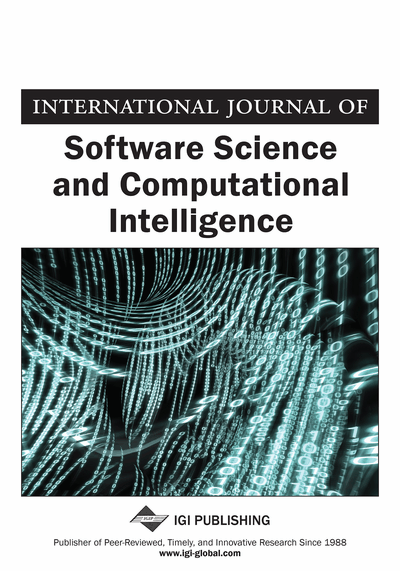# Information Processing Systems in UAV Based on Bayesian Filtering in Conditions of Uncertainty

DOI: 10.4018/IJSSCI.2020100103
OnDemand:
(Individual Articles)
Available
\$37.50
No Current Special Offers

## Abstract

In this article, the author considers the possibility of applying modern IT technologies to implement information processing algorithms in UAV motion control system. Filtration of coordinates and motion parameters of objects under a priori uncertainty is carried out using nonlinear adaptive filters: Kalman and Bayesian filters. The author considers numerical methods for digital implementation of nonlinear filters based on the convolution of functions, the possibilities of neural networks and fuzzy logic for solving the problems of tracking UAV objects (or missiles), the math model of dynamics, the features of the practical implementation of state estimation algorithms in the frame of added additional degrees of freedom. The considered algorithms are oriented on solving the problems in real time using parallel and cloud computing.
Article Preview
Top

## Introduction

The growth and development of the IT sphere and the power of the computers allows to apply more advanced methods of information processing in the sphere of UAV and air planes. The major algorithms for information processing in such the spheres are filtering and extrapolation of the parameters of the trajectories of objects upon their detection and tracking according to measurement data.

The greatest interest is represented by the implementation of numerical methods for nonlinear filtering of dynamic processes under conditions of uncertainty and insufficiency of a priori information since the quality of the state vector estimate directly affects the operation of a closed control system (Bain, A. & Crisan, D., 2009). To provide feedback in such a system, the control object is equipped with meters, with which you can determine the components of the state vector, while the measurements have limited accuracy. To improve the quality of state assessment, special algorithms are used that reduce the measurement noise, taking into account its parameters and the dynamics of the control object. In the case of nonlinear systems, for example, the methods of nonlinear Kalmanovskaya and Bayesian filtering, which are successfully used to solve problems of assessing the state of aircraft and other objects, are most popular.

Until recently, their practical value was small, since many algorithms were unrealizable due to the stringent requirements of real-time processing. Therefore, analytical approximations in the form of linear Kalman – Bucy filters, which make it possible to solve problems in real time, are most widely used in practice (Bergemann, K. & Reich, S., 2012).

The development of adaptive filtering methods for nonlinear dynamic processes described by a not fully known and time-varying mathematical model under the action of random perturbations with a changing family of probability densities is extremely important. One of the effective ways to solve the problem is the Bayesian algorithm. Since information on external influences is inaccurate for adaptive nonlinear filtering problems, such artificial intelligence methods as neural networks and fuzzy logic can serve as an excellent complement to traditional methods of statistical synthesis of adaptive nonlinear filtering systems. In this regard, the possibilities of using neural networks, fuzzy logic and knowledge bases when implementing numerical algorithms for adaptive nonlinear filtering to increase their efficiency are considered. Although an extended Kalman filter will be briefly considered here, we take the Bayesian approach to the synthesis of discrete nonlinear filtering algorithms, which allows us to completely solve the problem of both linear and nonlinear filtering in discrete time.

The extended Kalman filter uses the model of a stochastic continuous system:x˙ = (x, t) + w(1) and discrete measurements:zk = hk(x(tk)) + yk(2) where x is the state vector of the system, w is the noise of the system, yk is the measurement noise.

The task of filtering is to find a measurement function zk an estimate of the state vector of the x’k system that minimizes the variance of the error x'k-x (tk).

Let an estimate of the state vector xk− 1 be obtained at time tk− 1. Based on this estimate, the forecast of the state vector estimate xk (a priori estimate) is built, then zk measurements and correction of the estimate a priori based on x’k’ ’measurement results (a posteriori estimate) are carried out (Wilson, R. C. & Finkel, L., 2009). An estimate a priori of the state vector x’k ’is calculated by integrating the model Equation (3):

(3) with initial conditions x’(t) = x’k-1, x’(0) = x0.

The a priori estimate for the covariance error matrix for the linearized equations in P’k increments is calculated as (4), (5), (6):Pk’= φPk’’-1φT +Q(4)

(5) φ = E + Fδt(6) with the initial conditions P’(t) = Pk’’−1, P(0) = P0. Where, Q is the covariance matrix of the noise of the system (Doucet, A. & Johansen, A., 2009). A posteriori estimate for the state vector and covariance matrix errors are constructed as follows:x’k’’ = x’k’ + Kk(Zk – Hkx’k’)(7) Pk’’ = (I − KkHk) Pk’(8)where:

• R –covariance matrix of noise measurements

## Complete Article List

Search this Journal:
Reset
Volume 16: 1 Issue (2024): Forthcoming, Available for Pre-Order
Volume 15: 1 Issue (2023)
Volume 14: 4 Issues (2022): 1 Released, 3 Forthcoming
Volume 13: 4 Issues (2021)
Volume 12: 4 Issues (2020)
Volume 11: 4 Issues (2019)
Volume 10: 4 Issues (2018)
Volume 9: 4 Issues (2017)
Volume 8: 4 Issues (2016)
Volume 7: 4 Issues (2015)
Volume 6: 4 Issues (2014)
Volume 5: 4 Issues (2013)
Volume 4: 4 Issues (2012)
Volume 3: 4 Issues (2011)
Volume 2: 4 Issues (2010)
Volume 1: 4 Issues (2009)
View Complete Journal Contents Listing